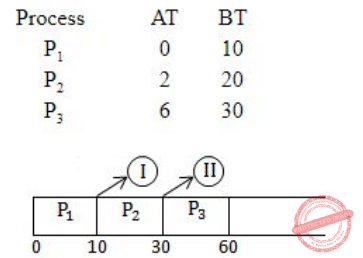# GATE2006-06, ISRO2009-14

8.3k views

Consider three CPU-intensive processes, which require $10$, $20$ and $30$ time units and arrive at times $0$, $2$ and $6$, respectively. How many context switches are needed if the operating system implements a shortest remaining time first scheduling algorithm? Do not count the context switches at time zero and at the end.

1. $1$
2. $2$
3. $3$
4. $4$

edited
0

@MiNiPanda

https://gateoverflow.in/885/gate2006-06-isro2009-14?show=220079#a220079

Processes execute as per the following Gantt chartSo, here only $2$ switching possible (when we did not consider the starting and ending switching )

now here might be confusion that at $t= 2 \ \ p1$ is preempted and check that available process have shortest job time or not, but he did not get anyone so it should not be consider as context switching.(same happened at $t=6$)

edited
–3

P1------>OS Scheduler--------->p2--------->OS scheduler--------->p3

and hence, Shouldn't there be 4 context switches?

0
4 in case of if we count both first and last context switching
0
Please just clear me... current process execution is finished and processor is going for another process is it a context switch? Is the cpu stores any context about the finished process?
3

According to Galvin,0

@tusharp

Here at time t=2 and t=6 context switch doesn't happens BUT preemption will be there right ?? as Scheduler will be loaded to decide which one load next ??

0
Can we say that in non preemptive scheduling if number of processes are n then number of Context switch will be n-1 if we exludee first and last ones?
3

Please just clear me... current process execution is finished and processor is going for another process is it a context switch? Is the cpu stores any context about the finished process?

Yes, it's a context switch, because the state of the process will change to terminated and it'll be saved in the PCB

0
switching of cpu from one process to another(in any case) is context switch, be it preemption or process completion or I/O.
0

Bro, you are counting one context switch as twice (you divided context switch process in two half and you are counting each half as one context switch, it is not fair)

0
Meghna, that is why initial and last CS is ignored.

So only 2
Only two switches wil be required after process 1 and 2 complete.Option B.

0

when execution of one process is completed and other process is scheduled , Is it called Context switch ?

I think , It is not Context switch, as according to wiki

In computing, a context switch is the process of storing the state of a process or of a thread, so that it can be restored and execution resumed from the same point later. This allows multiple processes to share a single CPU, and is an essential feature of a multitasking operating system.

As we are not storing results od earlier process for later execution. If there are any results produced by some operation within process or program , It is responsibility of program (or process when it running) to store them back.

For example : when C program to add two number gets converted to Assembly language code , It also has instruction to store result of addition back into memory.

Hence answer to this previous year gate question must be 0

0
@Headshot I also had this doubt while solving but none of the options matched :/
1 voteTotal no.of context switches is 2.

1
Correct

## Related questions

1
4.2k views
Consider the following code written in a pass-by-reference language like FORTRAN and these statements about the code. subroutine swap(ix,iy) it = ix L1 : ix = iy L2 : iy = it end ia = 3 ib = 8 call swap (ia, ib+5) print *, ia, ib end S1: The compiler will generate ... and 8 S5: The program will print 13 and -2 Exactly the following set of statement(s) is correct: S1 and S2 S1 and S4 S3 S1 and S5
Consider the polynomial $p(x) = a_0 + a_1x + a_2x^2 + a_3x^3$ , where $a_i \neq 0$, $\forall i$. The minimum number of multiplications needed to evaluate $p$ on an input $x$ is: 3 4 6 9
A CPU has $24$-$bit$ instructions. A program starts at address $300$ (in decimal). Which one of the following is a legal program counter (all values in decimal)? $400$ $500$ $600$ $700$
Consider three processes, all arriving at time zero, with total execution time of $10$, $20$ and $30$ units, respectively. Each process spends the first $\text{20%}$ of execution time doing I/O, the next $\text{70%}$ of time doing computation, and the last $\text{10%}$ of time doing ... . For what percentage of time does the CPU remain idle? $\text{0%}$ $\text{10.6%}$ $\text{30.0%}$ $\text{89.4%}$